1. # Implementing Assignment

Implementing Assignment

The operator= function provides a way to overload the assignment operator (=). Why would you want to do this? Suppose you have a class that contains a date and a time, and you want to make sure that these member variables hold the date and the time when the object is created or assigned to. If you don’t implement operator=, the existing values will be copied straight from the right side over to the left side. By implementing the operator, you can customize the copy operation and ensure that the date and the time are updated when the assignment is performed.

The following short exercise shows you how to implement operator= for the Dbl struct.

1.    Using the same project as in the previous exercises, add three data members to represent the time. Add the following line after the declaration of the val variable:

// creation or assignment time

double hr, min, sec;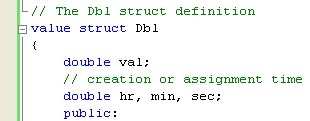2.        In addition, alter the constructor so that the time fields are initialized to the current time. The new constructor should read:

Dbl(double v)

{

val = v;

// Create new date and time fields

DateTime dt = DateTime::Now;

hr = dt.Hour;

min = dt.Minute;

sec = dt.Second;

}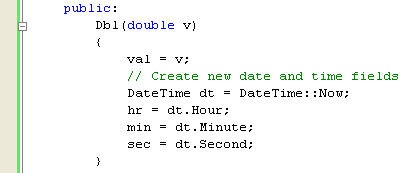The constructor uses a System::DateTime object to hold the current time; this object is initialized by a call to the static DateTime::Now property. The individual fields are then saved into the appropriate members of the object.

3.        Now add the assign function to the Dbl struct.

// The assignment operator for the Dbl struct

static Dbl op_Assign(Dbl& lhs, const Dbl& rhs)

{

// Copy over the value

lhs.val = rhs.val;

// Create new date and time fields

DateTime dt = DateTime::Now;

lhs.hr = dt.Hour;

lhs.min = dt.Minute;

lhs.sec = dt.Second;

return lhs;

}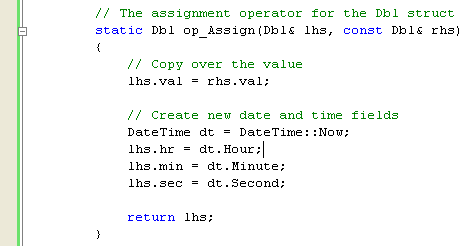You’ll notice several things about this function. First is the fact that it uses references for the arguments. Passing values by reference does not cause a copy to be made. Instead, the name you use in the function is a link back to the argument that was passed in. Passing in values by using references is essential when you think about how assignment has to work. Let’s say you code a line like this:

obj2 = obj1;

You want obj2 to contain the values from obj1 when the function returns. If you pass the arguments by value, without the &, any changes to lhs will be lost because this local variable ceases to exist at the end of the function. Making the arguments to the function references provides a link back to the value that was passed in, so changes made to lhs will be persistent. The rhs (right hand side) argument is made a const reference because you don’t want to make changes to the right side and the keyword const ensures that you can’t. The val member is copied over, and then a new set of time members is generated so that the object will reflect the time it was assigned, not the original time it was created.

To test the changes, you have to arrange some sort of time delay between creating the object and assigning to it so that you can see that the times are different. The easiest way to do this is to use the static Sleep method from the System::Thread class, so add the following code to your main() method:

// Create two objects

Dbl one(0.0);

Dbl two(10.0);

// Sleep for five seconds

Console::WriteLine("Wait... ");

// Do an assignment

one = two;

4.  The best way to see the result is to run the code under the debugger. Place a breakpoint in the code on the line where the one object is created by clicking in the gray margin to the left of the code. You’ll see a red dot appear, indicating an active breakpoint.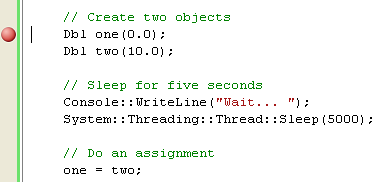5.  Run the program by pressing F5, and the code runs until the breakpoint is reached. Press F10 to single-step over the creation of the one object. Now look in the Locals window, and click the plus sign (+) to the left of one to expand it. You see the time values assigned to the object.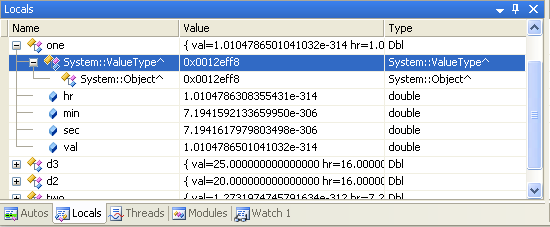6.  Now press F10 to single-step through the code, past the call to Sleep. When you pass the assignment, you see that the data members in one change; val takes on the value of the val member from two, while the time reflects the time when the assignment was done.7.        Build and run your program. The output should be something shown below.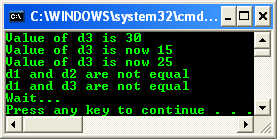Let recap, the assignment operator (=) is, strictly speaking, a binary operator. Its declaration is identical to any other binary operator, with the following exceptions:

1. It must be a non-static member function. No operator= can be declared as a nonmember function.

2. It is not inherited by derived classes.

3. A default operator= function can be generated by the compiler for class types if none exists.

The following example illustrates how to declare an assignment operator:

// assignment.cpp

// Compile with /clr

#include "stdafx.h"

using namespace System;

class Point

{

public:

// Right side is the argument.

Point &operator=(Point &);

int _x, _y;

};

// Define assignment operator.

Point &Point::operator=(Point &ptRHS)

{

_x = ptRHS._x;

_y = ptRHS._y;

// Assignment operator returns left side.

return *this;

}

int main()

{

Point p1, p2, p3;

p1._x = 3;

p2._y = 4;

p1 = p2 = p3;

return 0;

}

// no output.

Note that the supplied argument is the right side of the expression. The operator returns the object to preserve the behavior of the assignment operator, which returns the value of the left side after the assignment is complete. This allows writing statements such as:

pt1 = pt2 = pt3;